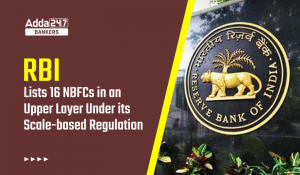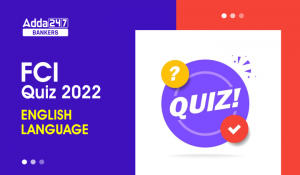Latest Banking jobs   »   Reasoning Ability Quiz For FCI Phase...

# Reasoning Ability Quiz For FCI Phase I 2022- 14th September

Directions (1-3): Read the following information carefully and answer the given questions.
D, L, N, X, W and V are six friends. Each of them has different weight. L is heavier than only N. W is lighter than only two people. V is not the heaviest. The difference between the weight of third lightest and the lightest person is 12kg. D is heavier than W but is not the heaviest. The weight of V is 70 kg.

Q1. How many persons are heavier than V?
(a) Four
(b) Three
(c) Two
(d) One
(e) None of these

Q2. Which of the following statement is true if weight of L is 66 kg?
(a) The difference between the weight of V and L is 6 kg.
(b) Weight of W is 65 kg.
(c) Weight of D is 24 kg.
(d) The difference between the Weight of L and N is 8 kg.
(e) None is true

Q3. What could be the possible Weight of W?
(a) 62 kg
(b) 66 kg
(c) 80 kg
(d) 69 kg
(e) 59 kg

Q4. Rohit ranked 15th from the top and 23nd from the bottom in a class. How many students are there in the class?
(a) 38
(b) 39
(c) 37
(d) 36
(e) 40

Q5. Deepak ranked 31st from the bottom and 13th from the top among those who passed an exam. six boys did not participate in the exam and four failed in it. How many boys were there in the class?
(a) 54
(b) 55
(c) 52
(d) 53
(e) 51

Direction (6-8): Study the following information carefully and answer the questions given below:
There are six poles in a ground. All poles are different height. Pole T is taller than U. Q is taller than S but shorter R. U is not the shortest Pole. P is taller than U and Q, which is shorter than T. The third shortest pole is 40m. Pole R is not the tallest. Pole U is taller than S. Height of tallest pole is 80m. Height of pole T is a prime number. T is not taller than R.

Q6. Which of the following pole is tallest?
(a) U
(b) Q
(c) P
(d) T
(e) None of these

Q7. Which of the following may be the height of pole T?
(a) 63m
(b) 31m
(c) 55m
(d) 67m
(e) None of these

Q8. Which of the following pole is second shortest?
(a) T
(b) U
(c) S
(d) R
(e) None of these

Q9. Veer is 28th from the left end of a row and Aman is 22th from the right end of row. If they interchanged their positions then Aman ranks become 13th from right end. Find total number of persons in the row?
(a) 40
(b) 41
(c) 38
(d) 39
(e) None of these

Q10. Anurag ranked 42th from the bottom and 6th from the top among those who passed an exam. Seven boys did not participate in the exam and three failed in it. How many boys were there in the class?
(a) 47
(b) 48
(c) 52
(d) 68
(e) 57

Q11. Shubham is 15th from the left end of a row of 45 boys and Akash is 21st from the right end in the same row. How many boys are there between them in the row?
(a) 11
(b) 9
(c) 12
(d) 15
(e) None of the above

Q12. In a row of student, Ananya is 14th from the right end and Bhanu is 12th from the left end. If in this row Bhanu is 20th from the right, then what is the position of Ananya from the left?
(a) 10
(b) 9
(c) 20
(d) 18
(e) None of the above

Directions (13-15): Study the following information carefully and answer the question given below.
Six boxes are placed in a vehicle in a horizontal arrangement. Each box has different weight. Box A is heavier than C but lighter than B. Weight of box F is an odd number. Box D is heavier than Box F but not the heaviest. Box C is lighter than D. Box F is heavier than box E, which is not the second lightest box. A is not second heaviest box. Weight of second lightest box is 56kg.

Q13. If the weight of A is 66kg, then what may be the weight of box F?
(a) 68
(b) 75
(c) 63
(d) 58
(e) None of these

Q14. Which of the following box is heaviest?
(a) F
(b) B
(c) A
(d) C
(e) None of these

Q15. Box E is heavier than how many boxes?
(a) Three
(b) One
(c) Two
(d) None
(e) More than Three

Solutions

S1. Ans. (b)
Sol. X > D > W > (70kg) V > L > N(58kg)

S2. Ans. (d)
Sol. X > D > W > (70kg) V > L > N(58kg)

S3. Ans. (c)
Sol. X > D > W > (70kg) V > L > N(58kg)

S4. Ans(c)
Sol. Number of students in the class= 15+23-1=37

S5. Ans(d)
Sol. Number of boys who passed = (31 + 13 – 1) = 43
∴ Total number of boys in the class = (43 + 6 + 4) = 53

S6. Ans. (c)
Sol. P (80) > R > T > Q (40) > U > S

S7. Ans. (d)
Sol. P (80) > R > T > Q (40) > U > S

S8. Ans. (b)
Sol. P (80) > R > T > Q (40) > U > S

S9. Ans. (a)
Sol. Total number of persons in the row= (28+13-1) = 40

S10. Ans. (e)
Sol. Number of boys who passed = (42 + 6 – 1) = 47
∴ Total number of boys in the class = (47 + 7 + 3) = 57

S11. Ans.(b)
Sol. Position of Shubham from right end= 45-(15-1) = 31
Position of Akash from right end= 21
So, no. of boys between them= 9

S12. Ans.(d)
Sol. Total number of students in row =12+20-1= 31
Position of Ananya from left end= 31-(14-1) = 18

S13. Ans. (c)
Sol. B > D > A > F > C (56)> E

S14. Ans. (b)
Sol. B > D > A > F > C (56)> E

S15. Ans. (d)
Sol. B > D > A > F > C (56)> E#### Congratulations!Download Hindu Review of October 2021: Free PDFIncorrect details? Fill the form again here

Download Hindu Review of October 2021: Free PDF

Thank You, Your details have been submitted we will get back to you.
•General-Studies Quiz For FCI Phase I 202...
•World Teacher's Day 2022, Theme, History...
•Govt plans to set up 100 labs for 5G tec...
•RBI Lists 16 NBFCs in Upper Layer Under ...
•English Quizzes For FCI Phase 1 2022- 3r...
•Quantitative Aptitude Quiz For FCI Phase...